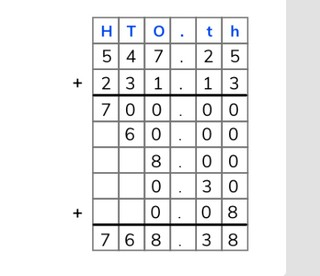Partial sums addition with decimal numbers to the hundredths place

# Partial sums addition with decimal numbers to the hundredths place

Partial sums addition with decimal numbers to the hundredths place8,000 schools use Gynzy92,000 teachers use Gynzy1,600,000 students use Gynzy

## General

Students learn to add decimal numbers to the hundredths place using partial sums.

5.NBT.B.7

## Relevance

Using partial sums to add decimal numbers allows you to add numbers that are more difficult to add in one step in your head. You can use this to easily add multiple decimal numbers together.

## Introduction

The interactive whiteboard shows two receipts, each showing the purchase of two products. The students must add the cost of the products together and calculate the total price of purchasing both objects.

## Development

Explain to students that when adding using partial sums that you write the numbers one below the other. Then you add from left to right. Tell students that you write the largest number on the top and the smaller number on the bottom. In this case, you start adding at the tens. Add these together. Then you add the ones, the tenths, and hundredths. Write all of the partial sums in the boxes and then add the partial sums together to find the total sum which you write at the bottom of the boxes. Show students a problem in which the problem is written in but where they need to write in the partial sums. Ask students to write down the partial sums on a sheet of paper and hold them up so that they can be checked. Next show three problems that students must solve using partial sums where the problems have already been written into the boxes. Then have students complete an entire problem including writing the problems into the boxes. At the end challenge students with a problem which has three numbers.

Check that students have understood addition with decimal numbers to the hundredths place using partial sums by asking the following questions/giving the following tasks:
- Why is it useful to be able to add using partial sums?
- Where do. you always start when adding with partial sums?
- Solve the following problems using partial sums: 343.25 + 121.25 = ?, 654.35 + 231.56 = ?

## Guided practice

Students are given addition problems with decimal numbers in which they are asked to determine the partial sums, problems in which they must write in the addition problem, and problems in which they must complete all of the steps for adding decimal numbers using partial sums.

## Closing

Discuss with students that it is important to be able to add decimal numbers to the hundredths place using partial sums because it makes adding decimal numbers (and larger decimal numbers) easier. Check that students know that when using partial sums that they must add from left to right. The interactive whiteboard shows three receipts, each with three purchases. Students must add the purchases together and determine the total cost of the objects.

## Teaching tips

Students who have difficulty adding decimal numbers using partial sums can be supported by spending extra time on the decimal place value chart. Make sure that they understand that they are solving each addition problem per place value and then finally adding all of them together for the final total.

### The online teaching platform for interactive whiteboards and displays in schools

• Save time building lessons

• Manage the classroom more efficiently

• Increase student engagement